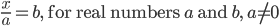# Definition | Equation Concepts | Linear Equation

This is a collection of definitions related to equations and similar topics. This includes general definitions for equations, as well as terms related to parts of an equation, different types of equations, and characteristics of equations.

### To see the complete collection of definitions, click on this link.

The following section provides additional information on solving one- and two-step equations.

## Solving One-Step Equations

A one-step equation can literally be solved in one step. This is because the equation is written in a form such that an inverse operation is enough to solve it. Here are the different forms a one-step equation can take:

 Equation Type Inverse Operation Example Addition Subtraction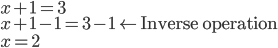Subtraction Addition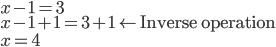Multiplication Division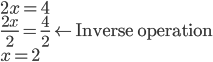Division MultiplicationWith these four basic cases, there are a number of variations, depending on the numbers involved. The simplest types of these equations involve positive whole numbers. But these equations can involve integers and rational numbers.

The general form of each of the four types of basic one-step equations are summarized here.

## Solving Two-Step Equations

You saw that with one-step equations, the “one step” involved one inverse operation. With two-step equations, there are two inverse operations involved in solving the equation. But this extra step introduces many more types of equations to solve, beyond the basic four from one-step equations.

There are 16 basic types of two-step equations that involve different combinations of the four basic operations.

 Addition Subtraction Multiplication Division Addition AA AS AM AD Subtraction SA SS SM SD Multiplication MA MS MM MD Division DA DS DM DD

These 16 basic examples are summarized in the table below, where we show an example of such an equation using numbers, then followed by a general form of the equation using variables and constant terms.

 Equation Type Inverse Operations Example General Form Addition and Addition Subtraction and Subtraction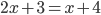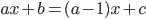Addition and Subtraction Subtraction and Addition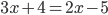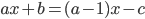Addition and Multiplication Subtraction and Division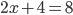Addition and Division Subtraction and Multiplication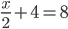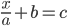Subtraction and Addition Addition and Subtraction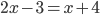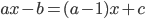Subtraction and Subtraction Addition and Addition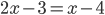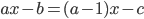Subtraction and Multiplication Addition and Division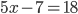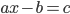Subtraction and Division Addition and Multiplication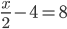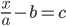Multiplication and Addition Division and Subtraction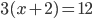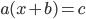Multiplication and Subtraction Division and Addition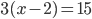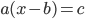Multiplication and Multiplication Division and Division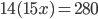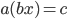Multiplication and Division Division and Multiplication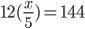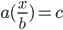Division and Addition Multiplication and Subtraction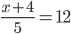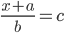Division and Subtraction Multiplication and Addition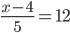Division and Multiplication Multiplication and Division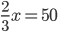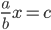Division and Division Multiplication and Multiplication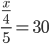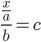These 16 basic two-step equations come in different forms depending on the sign of the numbers and whether the numbers are integers or rational numbers. The simplest types of these equations involve positive whole numbers.

 Equation Type General Form Addition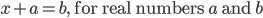Subtraction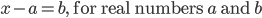Multiplication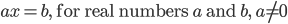Division